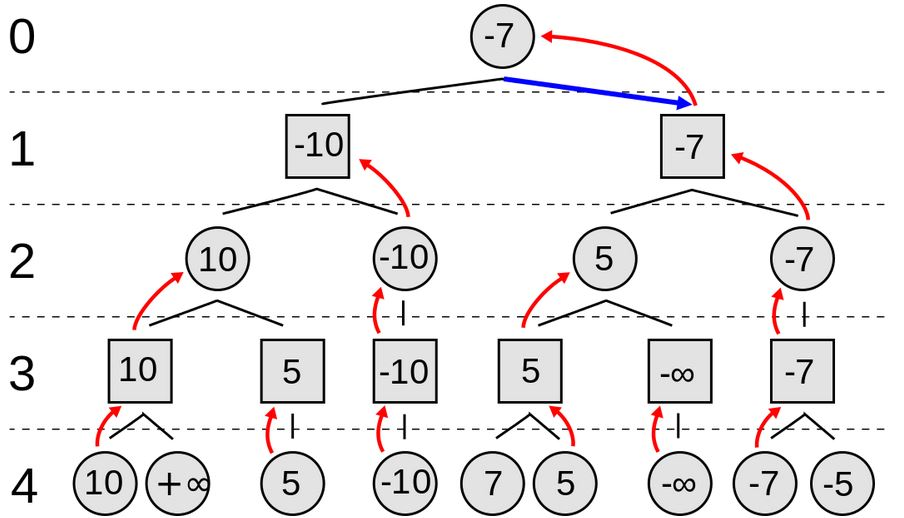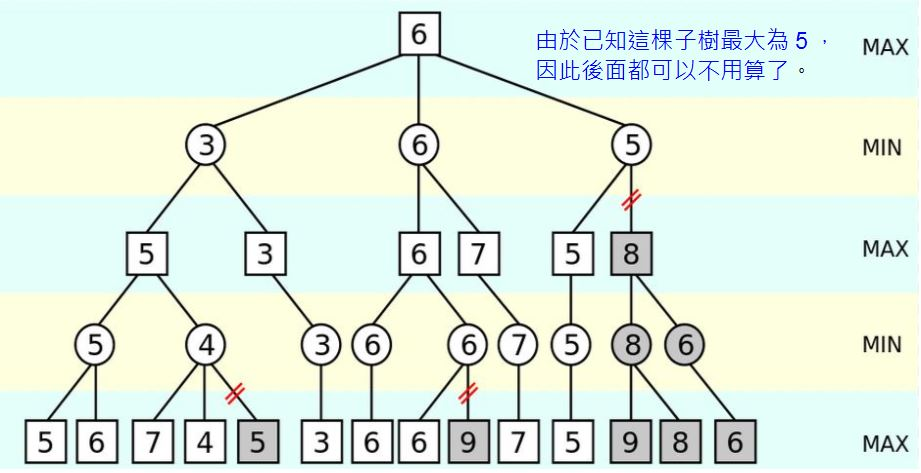# 程式人雜誌 -- 2014 年 7 月號 (開放公益出版品)

## 電腦下棋的關鍵： Min-Max 對局搜尋與 Alpha-Beta 修剪算法

### Min-Max 對局搜尋法``````function minimax(node, depth, maximizingPlayer)
if depth = 0 or node is a terminal node
return the heuristic value of node
if maximizingPlayer
bestValue := -∞
for each child of node
val := minimax(child, depth - 1, FALSE))
bestValue := max(bestValue, val);
return bestValue
else
bestValue := +∞
for each child of node
val := minimax(child, depth - 1, TRUE))
bestValue := min(bestValue, val);
return bestValue

(* Initial call for maximizing player *)
minimax(origin, depth, TRUE)``````

### Alpha-Beta 修剪法「Alpha-Beta 修剪法」其實是「Min-Max 對局搜尋法」的一個修改版，主要是在 Min-Max 當中加入了 α 與 β 兩個紀錄值，用來做為是否要修剪的參考標準，演算法如下所示。

``````function alphabeta(node, depth, α, β, maximizingPlayer)
if depth = 0 or node is a terminal node
return the heuristic value of node
if maximizingPlayer
for each child of node
α := max(α, alphabeta(child, depth - 1, α, β, FALSE))
if β ≤ α
break (* β cut-off *)
return α
else
for each child of node
β := min(β, alphabeta(child, depth - 1, α, β, TRUE))
if β ≤ α
break (* α cut-off *)
return β

(* Initial call for maximizing player *)
alphabeta(origin, depth, -∞, +∞, TRUE)``````

### 參考文獻

【本文由陳鍾誠取材並修改自 維基百科，採用創作共用的 姓名標示、相同方式分享 授權】